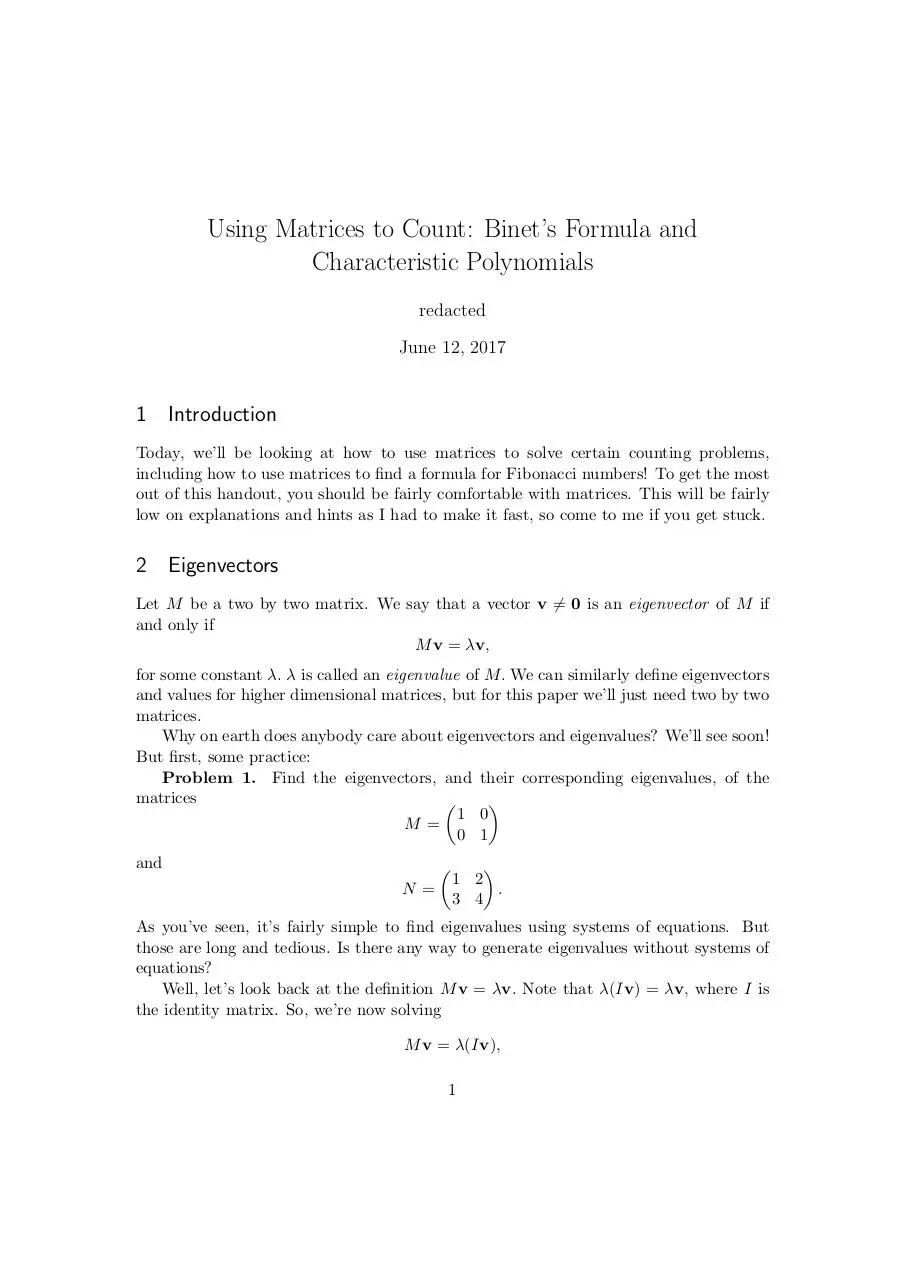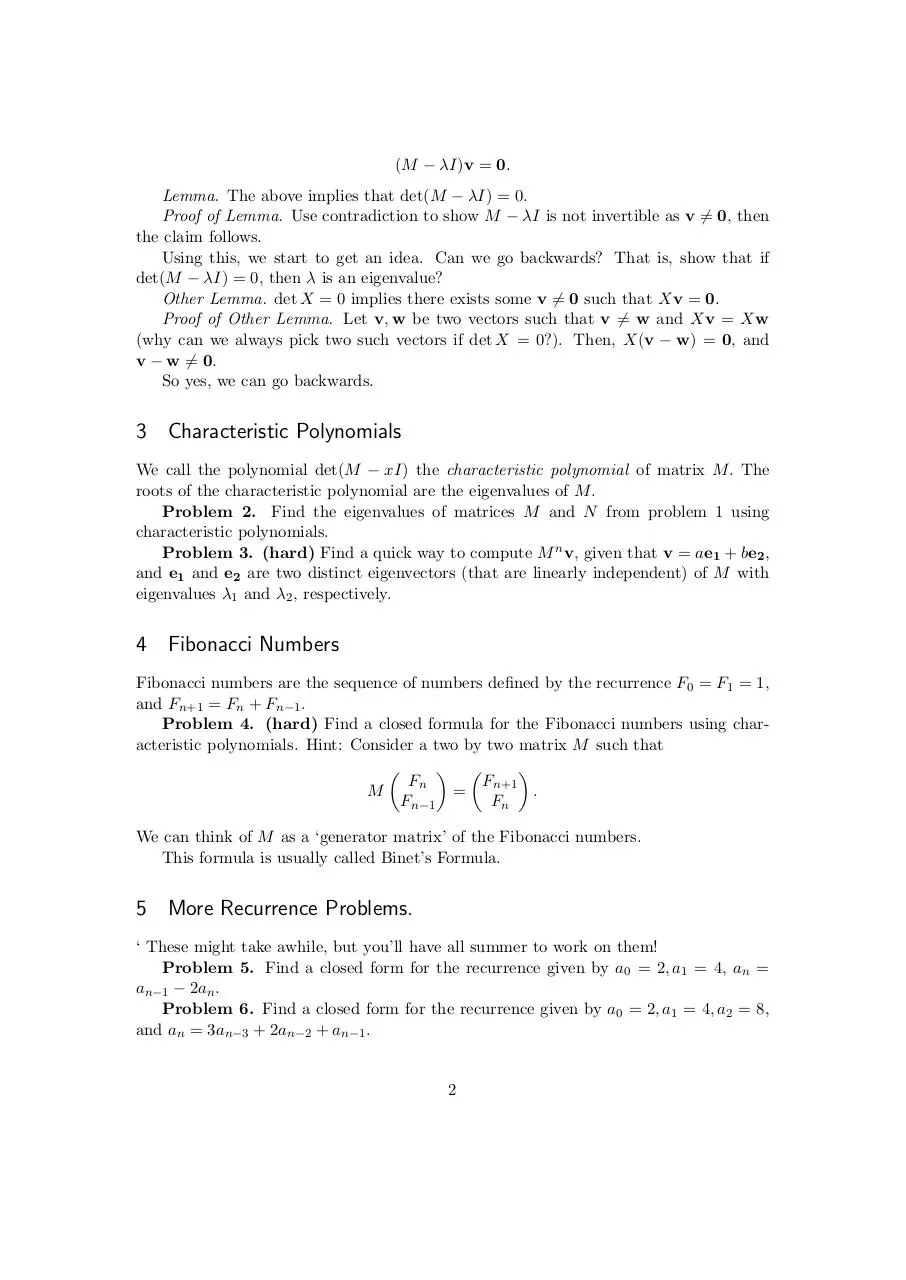# matrices and fibonacci .pdf

### File information

This PDF 1.5 document has been generated by TeX / MiKTeX pdfTeX-1.40.17, and has been sent on pdf-archive.com on 15/06/2017 at 01:06, from IP address 108.69.x.x. The current document download page has been viewed 1464 times.
File size: 146.07 KB (3 pages).
Privacy: public file

### Document preview

Using Matrices to Count: Binet’s Formula and
Characteristic Polynomials
redacted
June 12, 2017

1 Introduction
Today, we’ll be looking at how to use matrices to solve certain counting problems,
including how to use matrices to find a formula for Fibonacci numbers! To get the most
out of this handout, you should be fairly comfortable with matrices. This will be fairly
low on explanations and hints as I had to make it fast, so come to me if you get stuck.

2 Eigenvectors
Let M be a two by two matrix. We say that a vector v 6= 0 is an eigenvector of M if
and only if
M v = λv,
for some constant λ. λ is called an eigenvalue of M. We can similarly define eigenvectors
and values for higher dimensional matrices, but for this paper we’ll just need two by two
matrices.
Why on earth does anybody care about eigenvectors and eigenvalues? We’ll see soon!
But first, some practice:
Problem 1. Find the eigenvectors, and their corresponding eigenvalues, of the
matrices


1 0
M=
0 1
and


N=


1 2
.
3 4

As you’ve seen, it’s fairly simple to find eigenvalues using systems of equations. But
those are long and tedious. Is there any way to generate eigenvalues without systems of
equations?
Well, let’s look back at the definition M v = λv. Note that λ(Iv) = λv, where I is
the identity matrix. So, we’re now solving
M v = λ(Iv),
1

(M − λI)v = 0.
Lemma. The above implies that det(M − λI) = 0.
Proof of Lemma. Use contradiction to show M − λI is not invertible as v 6= 0, then
the claim follows.
Using this, we start to get an idea. Can we go backwards? That is, show that if
det(M − λI) = 0, then λ is an eigenvalue?
Other Lemma. det X = 0 implies there exists some v 6= 0 such that Xv = 0.
Proof of Other Lemma. Let v, w be two vectors such that v 6= w and Xv = Xw
(why can we always pick two such vectors if det X = 0?). Then, X(v − w) = 0, and
v − w 6= 0.
So yes, we can go backwards.

3 Characteristic Polynomials
We call the polynomial det(M − xI) the characteristic polynomial of matrix M. The
roots of the characteristic polynomial are the eigenvalues of M.
Problem 2. Find the eigenvalues of matrices M and N from problem 1 using
characteristic polynomials.
Problem 3. (hard) Find a quick way to compute M n v, given that v = ae1 + be2 ,
and e1 and e2 are two distinct eigenvectors (that are linearly independent) of M with
eigenvalues λ1 and λ2 , respectively.

4 Fibonacci Numbers
Fibonacci numbers are the sequence of numbers defined by the recurrence F0 = F1 = 1,
and Fn+1 = Fn + Fn−1 .
Problem 4. (hard) Find a closed formula for the Fibonacci numbers using characteristic polynomials. Hint: Consider a two by two matrix M such that

 

Fn
Fn+1
M
=
.
Fn−1
Fn
We can think of M as a ‘generator matrix’ of the Fibonacci numbers.
This formula is usually called Binet’s Formula.

5 More Recurrence Problems.
‘ These might take awhile, but you’ll have all summer to work on them!
Problem 5. Find a closed form for the recurrence given by a0 = 2, a1 = 4, an =
an−1 − 2an .
Problem 6. Find a closed form for the recurrence given by a0 = 2, a1 = 4, a2 = 8,
and an = 3an−3 + 2an−2 + an−1 .

2

Problem 7. The formula for Fibonacci numbers uncovered in the last section is
pretty sucky. There’s a cooler formula using the floor function. Try to find it.
Problem 8. Try finding a matrix M whose eigenvectors are all linearly dependent.
What kind of interesting properties does it have? Can you find any recurrences that
relate to it?

6 Conclusion
major in math, so I really hope this interested you.

3matrices and fibonacci.pdf (PDF, 146.07 KB)

### Share on social networks

#### HTML Code

Copy the following HTML code to share your document on a Website or Blog